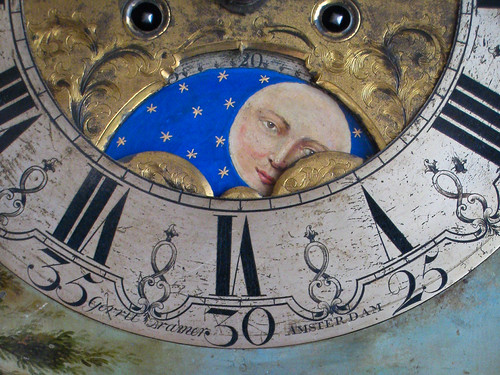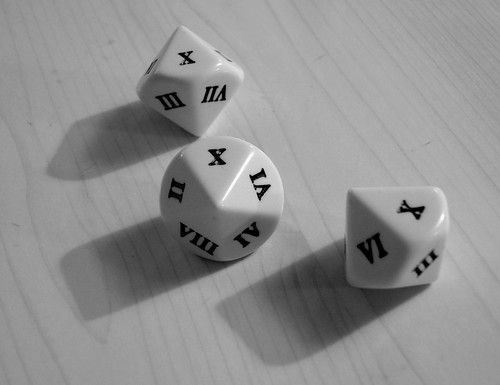# Explorations of Number Systems Support Strong Mathematical Skills

### Explorations of Number Systems Support Strong Mathematical SkillsBy exploring number systems, families can help children to solidify their own understanding of the numerals and place value of our own familiar base ten number system. From binary code and Roman numerals to a lost 13th century cipher and a zero-less system from 300 BC, number systems from around the world can lead to fascinating explorations of numerical representations and place value.

Mastering our ten-digit number system can present challenge aplenty for children – even after they’ve mastered basic numeracy skills like one-to-one correspondence and cardinality, understanding place value and the ways in which it influences the function of each of the four mathematical operations can take years of experience and practice. In order to support mathematicians of almost any age in strengthening their understanding of numbers and place value, families can explore number systems and digits that are different from the base-ten system that we use. By learning how to write numbers using unfamiliar symbols and exploring the theory behind number systems using a base of fewer than ten digits.

The youngest of budding mathematicians may benefit most from exploring number systems that consist of ten digits, but do not use the same Arabic numerals that we use today. The Brahmi number system, developed in the third century BC, includes only nine digits but is still similar to our familiar number system.The only difference? The Brahmi digits only represent quantities from 1-9, and don’t include a zero! Eventually, the concept of zero was introduced, and the original Brahmi digits underwent a slow evolution through three more systems before arriving at the numeral set that we now use. Practicing writing familiar quantities using unfamiliar characters can help children to understand that quantities are concrete, no matter the means through which we record them. Intrepid mathematicians can even try to do some basic calculations in Brahmi or another number system from history!

Concepts relating to place value can be explored by examining the binary system, the language used in computer programming. As its name suggests, binary is a two-digit (or base two) number system made up only of the digits 1 and 0. As a result, binary can be used to represent quantities in a very similar way to our Arabic number system – but place value works very differently. Rather than having places for ones, tens, hundreds, and so on, the two-digit binary system has places for ones, twos, fours, eights, and so on – with each place being twice as large as the place before it.

Another way in which budding mathematicians can explore the binary system is by learning about Ba-Gua numbers, a Chinese system that’s actually part of feng shui. Made up of eight numerals, the ways in which Ba-Gua numbers are written is based in binary. Each digit is made up of a series of solid dashes (representing a 1) and broken dashes (representing a zero), thus producing a binary number equivalent to an amount equal to a digit from 0-7. There are many more layers to truly understanding Ba-Gua, but for children, the real challenge lies in learning to use Ba-Gua’s numerals with the place value rules of binary – a task that requires simultaneous processing of unfamiliar characters and an unfamiliar place value system.Further explorations of number systems can include learning to use both Roman numerals and the long-lost Middle Ages cipher of Agrippa. Roman numerals, derived from the ways in which numbers can be represented using the fingers on human hands, include numerical representations from I to MM – the meanings of which kids can discover by learning to write the numerals or by simply counting pigs. Agrippa’s cipher, on the other hand, is based on using a single vertical line to create single-character written representations of quantities up to 9,999. Created in Europe in the 13th century, the cipher was especially useful for categorizing manuscripts, as it ca generate very compact numerical labels for items. The cipher can be used as further practice in representing familiar quantities semi-abstractly, or can even be used within families as a secret code!

Follow your interests in number systems! Check out this post, 12 Mind Blowing Number Systems From Other Languages.

[Photo credits: (cc) Nathan Reading; (cc) James Bowe ]